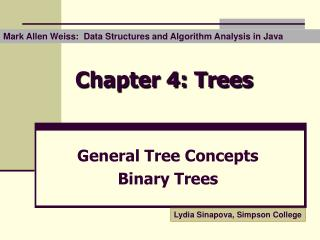DownloadDownload PresentationChapter 4: Trees

Chapter 4: Trees

Télécharger la présentationChapter 4: Trees

- - - - - - - - - - - - - - - - - - - - - - - - - - - E N D - - - - - - - - - - - - - - - - - - - - - - - - - - -
Presentation Transcript

1. Mark Allen Weiss: Data Structures and Algorithm Analysis in Java Chapter 4: Trees General Tree Concepts Binary Trees Lydia Sinapova, Simpson College

2. Trees • Definitions • Representation • Binary trees • Traversals • Expression trees

3. Definitions • tree - a non-empty collection of vertices & edges • vertex (node)- can have a name and carry other associatedinformation • path- list of distinct vertices in which successive vertices are connected by edges

4. Definitions • any two vertices must have one and only one path between them else its not a tree • a tree withN nodeshasN-1 edges

5. Definitions • root- starting point (top) of the tree • parent(ancestor) - the vertex “above” this vertex • child (descendent) - the vertices “below” this vertex

6. Definitions • leaves(terminal nodes) - have no children • level - the number of edges between this node and the root • ordered tree - where children’s order is significant

7. Definitions • Depth of a node- the length of the path from the root to that node • root: depth 0 • Height of a node- the length of the longest path from that node to a leaf • any leaf: height 0 • Height of a tree:The length of the longest path from the root to a leaf

8. Balanced Trees • the difference between the height of the left sub-tree and the height of the right sub-tree is not more than 1.

9. root E A R E Child (of root) S T A Leaves or terminal nodes M P L E Depth of T: 2 Height of T: 1 Trees - Example Level 0 1 2 3

10. Tree Representation Class TreeNode { Object element; TreeNode firstChild; TreeNode nextSibling; }

11. Example a b f e a g d c b e f g c d

12. S P O I N S M A Internal node External node D B N Binary Tree

13. L 0 L 1 L 2 L 3 Height of a Complete Binary Tree At each level the number of the nodes is doubled. total number of nodes: 1 + 2 + 22 + 23 = 24 - 1 = 15

14. Nodes and Levels in a Complete Binary Tree Number of the nodes in a tree with M levels: 1 + 2 + 22 + …. 2M = 2 (M+1) - 1 = 2*2M - 1 Let N be the number of the nodes. N = 2*2M - 1, 2*2M = N + 1 2M = (N+1)/2 M = log( (N+1)/2 ) N nodes : log( (N+1)/2 ) = O(log(N)) levels M levels: 2 (M+1) - 1 = O(2M ) nodes

15. Binary Tree Node Class BinaryNode { Object Element; // the data in the node BinaryNode left; // Left child BinaryNode right; // Right child }

16. C O T M E R U P L N A D Binary Tree – Preorder Traversal Root Left Right First letter - at the root Last letter – at the rightmost node

17. Preorder Algorithm preorderVisit(tree) { if (current != null) { process (current); preorderVisit (left_tree); preorderVisit (right_tree); } }

18. U P T O R E C M A D L N Binary Tree – Inorder Traversal Left Root Right First letter - at the leftmost node Last letter – at the rightmost node

19. Inorder Algorithm inorderVisit(tree) { if (current != null) { inorderVisit (left_tree); process (current); inorderVisit (right_tree); } }

20. D P N M A U C O L R T E Binary Tree – Postorder Traversal Left Right Root First letter - at the leftmost node Last letter – at the root

21. Postorder Algorithm postorderVisit(tree) { if (current != null) { postorderVisit (left_tree); postorderVisit (right_tree); process (current); } }

22. Expression Trees The stack contains references to tree nodes (bottom is to the left) 3 + * 1 2 2 3 1 + (1+2)*3 2 1 Post-fix notation:1 2 + 3 *

23. * 3 + 2 1 Expression Trees In-order traversal: (1 + 2) * ( 3) Post-order traversal: 1 2 + 3 *